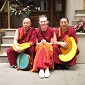# Extended rule

Extended rules in Map Editor

## Extended rule - Converting GMT Date time into CE(S)T and Vice Versa

//Extended rule - Converting GMT Date time into CE(S)T and Vice Versa

datetime d1,d2,d3;
integer dMonth,dDay,tZone,dYear;
String year,temp;
d1='';
d2='';
d3='';
year="";
dMonth=0;
dDay=0;
dYear=0;
tZone=0;

// let the date format be yyyy-mm-dd hh:mm:ss
//converting string to date format[#F is a field type is string]

d1=date("%Y-%m-%d %H:%M:%S", #F);
dMonth= get months (d1);
dYear= get years (d1);
ntoa(dYear,year);

temp=year+"-"+"03"+"-"+"31"+" 01:00:00";
d2=date("%Y-%m-%d %H:%M:%S",temp);
while(1)
do
begin
dDay=get days(d2);
if dDay=1 then break;
d2=d2<<days(-1);
end

temp=year+"-"+"10"+"-"+"31"+" 01:00:00";
d3=date("%Y-%m-%d %H:%M:%S",temp);
while(1)
do
begin
dDay=get days(d3);
if dDay=1 then break;
d3=d3<<days(-1);
end

//GMT to CE(S)T

if d1>d2 & d1<d3 then d1 = d1 << hours(2);
else d1 = d1 << hours(1);

//CE(S)T to GMT

//if d1>d2 & d1<d3 then d1 = d1 << hours(-2);
//else d1 = d1 << hours(-1);

strdate(d1,"%Y-%m-%d %H:%M:%S",#F);## Extended rule - get a system date in a map

System date, comparison of dates and adding dates to a field value of datetime type

// this extended rule explains how to take system date, compare it with date field from input data and also add some days to the field value
object sysDate;
object sysDateFormat;
string sysDateString;
datetime sysDate2;

sysDate = new("java.util.Date");
sysDateFormat = new("java.text.SimpleDateFormat","yyyyMMdd");
sysDateString = sysDateFormat.format(sysDate);

sysDate2 = date("%y%y%m%d",sysDateString);
if (#field_date < sysDate2) then
begin
messagebox("date older than system date",0);
#field_date = sysDate2 << days(7);
end
else
begin